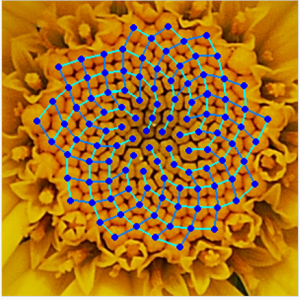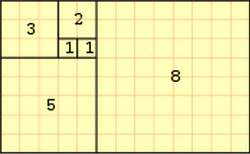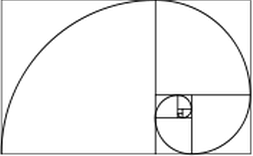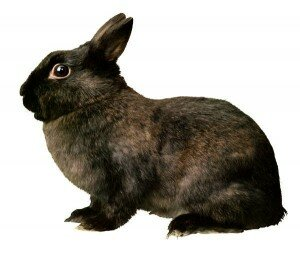# The Fibonacci SequenceFibonacci is a Number / Integer Sequence, that when applied in geometrical form, manifests in a Spiral as in that of a Pine Cone or a SeaShell.  The sequence was named after an Italian mathematician known as Leonardo of Pisa (or Leonardo de Fibonacci).  In 1202, he wrote a book called Liber Abaci in which he gives name to the number sequence.  There are historical examples of the sequence showing up in East Indian mathematics as well.

Add two consecutive numbers from the sequence to equal the next one following.  The basic sequence looks like this:

0,         1,            1,            2,            3,            5,            8,            13,            21,            34,            55,            89,            144,            233,            377, etc., etc.

That is:

0+1=1

1+1=2

2+1 (the “number” before)=3

3+2 (the number before)=5

5+3=8

8+5=13

13+8=21

34+21=55

55+34=89

89+34=144

144+233=377

etc., etc., etc.

In Spiritual Theory, Life must look back on itself before it can move forward.  In Relation to the human species, we must look back toward our Ancestors to learn Wisdom and give Gratitude to Life in the Present in order to move into the Future in the best way and in the Best Direction.  Because in theory the Spiral is not quantifiable in the concrete sense; i.e. it is a sequence that is Infinite (no final end number), mathematicians use straight lines around the spirals to give it as close to a concrete geometric equation as is possible.  Hence, the spiral looks like a spiral of expanding squares as shown here, and is known as the Golden Mean Ratio.In biological settings, The Fibonacci Sequence can be seen in the Spirals of the Pinecone, in the Branch growth pattern of trees, the mini-fruit pieces of the Pineapple, the Artichoke flower, a Fern during its uncurling, and Seashells.  The sequence can also be seen in Rabbit breeding patterns, and the family tree of Honeybees.

In relation to Rabbits, Fibbonaci posed this question during the middle ages:

Rabbits can mate at the age of one month, a pretty fast breeding cycle for an animal. If a rabbit population was ideal (though not biologically realistic), if one assumed that a new pair of rabbits (one male and one female) were in a field, and after the end of one month, that pair had another pair, and every pair had another pair, how many pairs would there be after one year?End of First Month:  1 New Pair

End of Second Month:  2 New Pairs

End of Third Month: The Original Female makes a Second Pair, Equaling 3 Pairs in the field

End of The Fourth Month:  First Female makes a Third Pair, The Female born in the Second Month makes Her First Pair, etc., Now Equaling 5 Pairs.

End of “n”th Month, Number of Pairs = The Number of New Pairs (= Number of Pairs in Month “n” – 2 + Number of Pairs alive in Previous Month “n” -1.

This is the “n”th Fibonacci Number, and it looks like this:

http://www.maths.surrey.ac.uk/hosted-sites/R.Knott/Fibonacci/fibnat.html#Rabbits

There are numerous other examples in nature shown in this site, as well as in class activities you can do to demonstrate the Fibonacci.

http://en.wikipedia.org/wiki/Fibonacci_number

And for more of our Fun Learning Math Games, you can visit here:

http://www.math-lessons.ca/activities/index.html